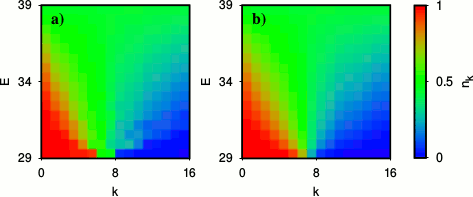Dynamical thermalization
of interacting fermionic atoms
in a Sinai-oscillator trap
by K.M.Frahm, L.Ermann and D.L.Shepelyansky
arXiv:1907.06711[cond-mat.quant-gas]Orbital occupation numbers n_k in the plane of energy E and orbital index k: a)result for exact eigenstates of Hamiltonian with Aberg parameter A=3.5; b) thermal Fermi-Dirac distibution (7 fermions of 16 orbitals).

 ` `Invited contribution to the Special Issue Cond. Matter MDPI in memory of Shmuel Fishman (Technion)Article download: MDPI published (here), arXiv-v1 (here)All figs in gzip format (here )Video example of time evolution (log-time scale) of density matrix from t/Delta-t=0 to 10^6 for 7 fermions (initially 2 excited), 16 orbitals and Aberg parameter A=3.5 (color shows density matrix; format avi, see details in README file) (video), ( README)Video of above example of time evolution (log-time scale) of spacial density from t/Delta-t=0 to 10^6 for 7 fermions (initially 2 excited), 16 orbitals and Aberg parameter A=3.5 (color shows wavefunction probability; format avi, see details in README file) (video), ( README)Video of above example of time evolution (log-time scale) of spacial density difference from t/Delta-t=0 to 10^6 for 7 fermions (initially 2 excited), 16 orbitals and Aberg parameter A=3.5 (color shows wavefunction probability; format avi, see details in README file) (video), ( README)Video of above example of time evolution (log-time scale) of density correlator from t/Delta-t=0 to 10^6 for 7 fermions (initially 2 excited), 16 orbitals and Aberg parameter A=3.5 (color shows wavefunction probability; format avi, see details in README file) (video), ( README)Videos for variety of cases at different parameters (avi-format, all files in one compressed directory (597Mb) (video), ( README)Related research on dynamical thermalization of Bose-Einstate condensate in a Sinai oscillator L.Ermann, E.Vergini and D.L.Shepelyansky Phys. Rev. A v.94, p.013618 (2016) (web site) This webpage is created at July 7, 2019 and is maintained by L.Ermann, K.M.Frahm and D.L.Shepelyansky